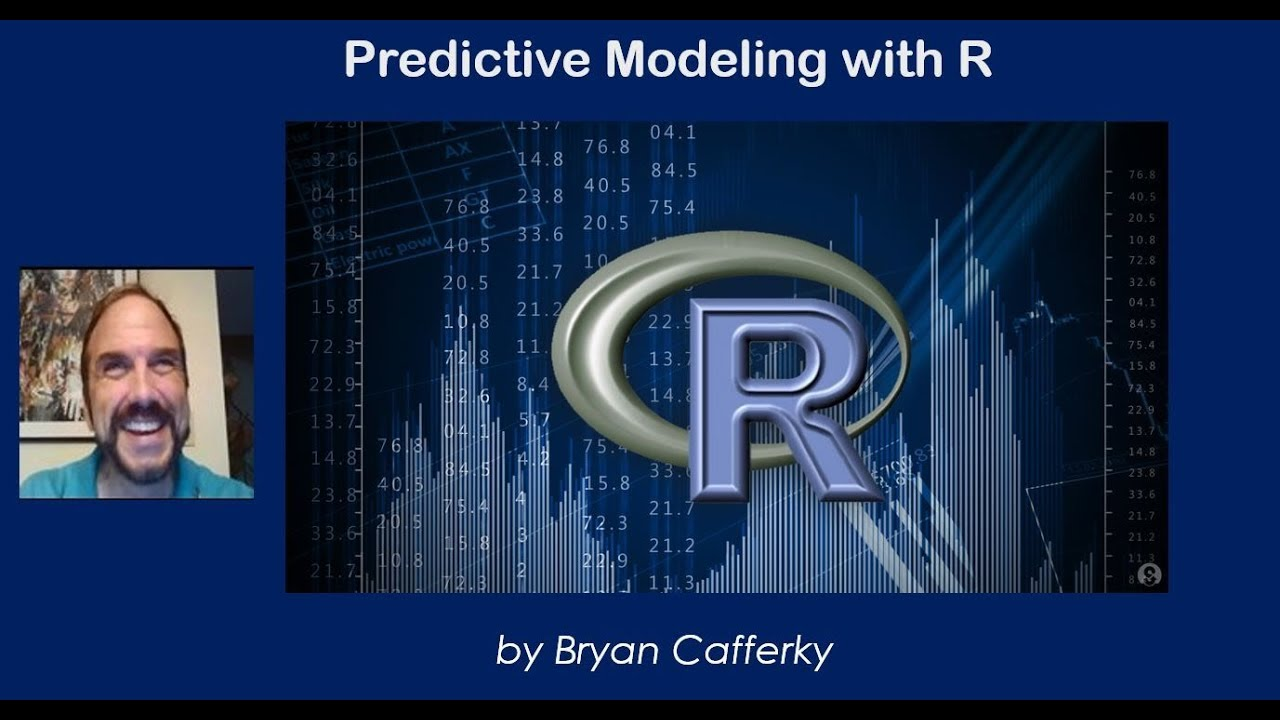# Understanding Predictive Modeling with RLearn what predictive modeling is and how to create your own models using the awesome R programming language. This video explains the concepts and demonstrates how to train and test a predictive.

Learn what predictive modeling is and how to create your own models using the awesome R programming language. This video explains the concepts and demonstrates how to train and test a predictive.

Content available at https://github.com/bcafferky/shared/tree/master/RPredictiveModeling

## R Programming Tutorial for Beginners | Learn the Basics of R Programming | R & RStudio Tutorial

👉 This video will introduce you to the R programming language, and will give you a hands-on overview of the statistical programming language R, one of the most important programming tools in data science..

## R Programming For Beginners-Full Course | Learn R in 3 Hours| R Language Tutorial

Great Learning brings you this tutorial on R Programming for Beginners to help you understand everything you need to know about this topic and getting started on the journey to learn about it well. This video starts with an introduction to R and its installation, followed by understanding the concepts of variables, data types and operators, etc. in R. Following this, we will understand data manipulation with dplyr and data visualization with ggplot2. Finally, we learn about neural networks! This video teaches R programming and its key functions and concepts with a case study, demonstrations & examples to help you get started on the right foot.

## How To Import Data Into R | R Programming Basics | R Programming For Beginners

This video on How to import data in R will make your understand how to load and read data from different file types. You will learn the R programming basics along with different packages and functions required to import data present in text, csv, excel and sas files. You will also look at how to write data from R to an excel file.

## R Programming Course

🔵 Intellipaat Data Science with R course: https://intellipaat.com/data-scientist-course-training/In this R Tutorial For Beginners video, you will learn r pr...

## Data Types In R

Data types are kept easy. Data types of R are quite different when we compare with other programming languages. Here, we’ll outline the data types of R.